# 1. A particle with a charge of +4.20 nC is in a uniform electric field E⃗  directed...

1. A particle with a charge of +4.20 nC is in a uniform electric field E⃗  directed to the negative x direction. It is released from rest, and after it has moved 6.00 cm , its kinetic energy is found to be 1.50×10−6 J . a. What work was done by the electric force? b. What was the change in electric potential over the distance that the charge moved? c. What is the magnitude of E? d. What was the change in potential energy of the charge?

2. A uniform electric field has magnitude E and is directed in the negative x direction. The potential difference between point a (at x= 0.70 m ) and point b (at x= 0.95 m ) is 300 V . a. Calculate the value of E. b. A negative point charge q=−0.200μC is moved from b to a. Calculate the work done on the point charge by the electric field.

3. A +2.30 μC point charge is sitting at the origin. a. What is the radial distance between the 500 V equipotential surface and the 1000 V surface? b. What is the distance between the 1000 V surface and the 1500 V surface?

4. The plates of a parallel-plate capacitor have a separation of 3.40 mm , and each has an area of 13.8 cm2 . Each plate carries a charge of magnitude 8.00×10−8 C . The plates are in vacuum. a. What is the capacitance? b. What is the potential difference between the plates? c. What is the magnitude of the electric field between the plates?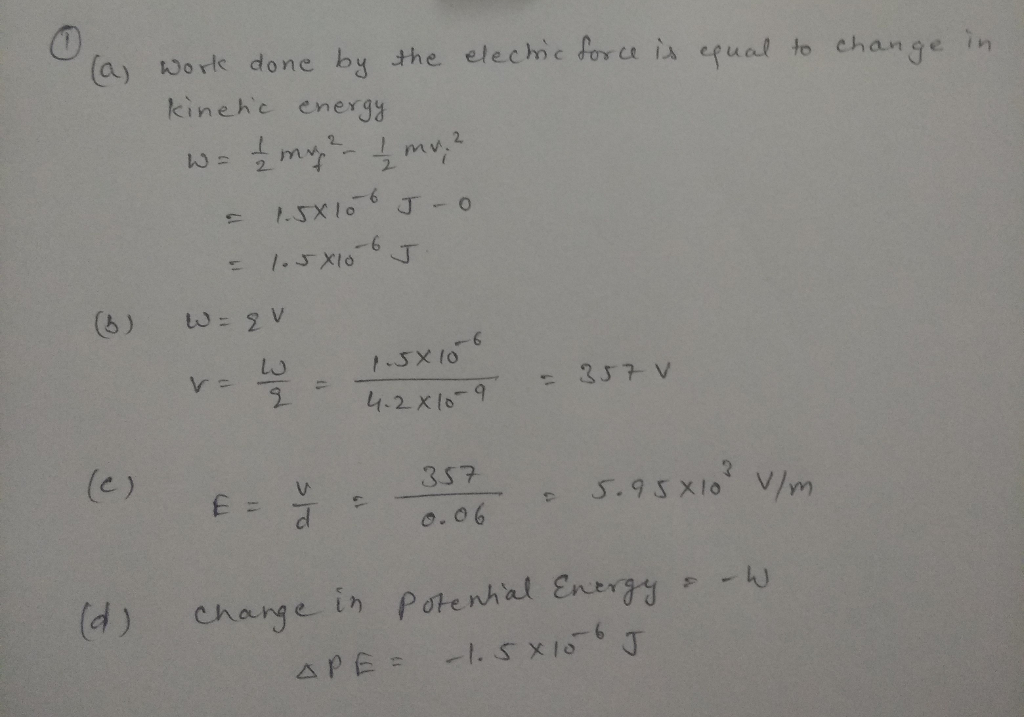#### Earn Coin

Coins can be redeemed for fabulous gifts.

Similar Homework Help Questions
• ### 13. I A particle with a charge of +4.20 nC is in a uniform electric field...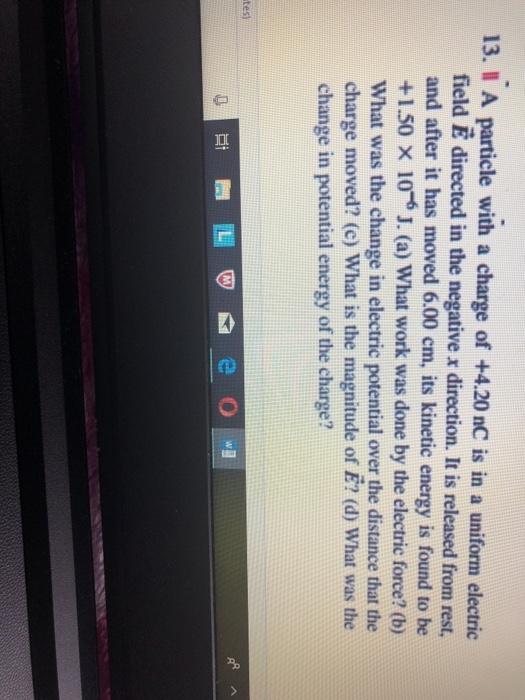13. I A particle with a charge of +4.20 nC is in a uniform electric field È directed in the negative x direction. It is released from rest, and after it has moved 6.00 cm, its kinetic energy is found to be + 1.50 × 10-6 J. (a) what work was done by the electric force? (b) What was the change in electric potential over the distance that the charge moved? (c) What is the magnitude of E? (d) What...

• ### Question 4: (10 marks) A uniform electric field has magnitude E and is directed in the...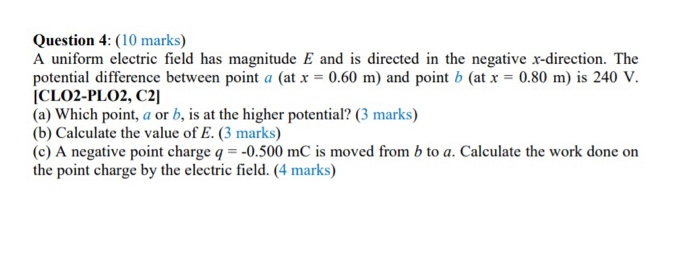Question 4: (10 marks) A uniform electric field has magnitude E and is directed in the negative x-direction. The potential difference between point a (at x = 0.60 m) and point b (at x = 0.80 m) is 240 V. (CLO2-PLO2, C2) (a) Which point, a or b, is at the higher potential? (3 marks) (b) Calculate the value of E. (3 marks) (c) A negative point charge q = -0.500 mC is moved from b to a. Calculate the...

• ### 1. A charge of 27.0 nC is placed in a uniform electric field that is directed...

1. A charge of 27.0 nC is placed in a uniform electric field that is directed vertically upward and that has a magnitude of 4.00×104 N/C . What work is done by the electric force when the charge moves... a. 0.500 m to the right b. 0.640 m upward; c. 2.20 m at an angle of 45.0 downward from the horizontal? 2. A potential difference of 5.00 kV is established between parallel plates in air. If the air becomes ionized...

• ### A uniform electric field has magnitude E and is directed in the negative i direction. The...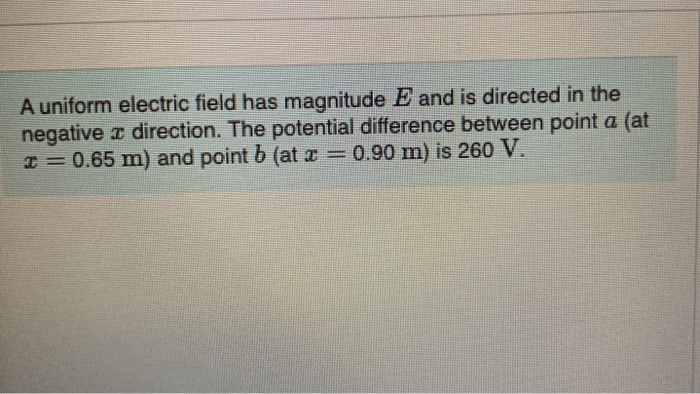A uniform electric field has magnitude E and is directed in the negative i direction. The potential difference between point a (at T= 0.65 m) and point b (at 2 = 0.90 m) is 260 V. Calculate the value of E. Express your answer in newtons per coulomb. EVO AO O ? E- N/C Submit Request Answer Part C C is moved from b to a. Calculate the work done on the point charge by the electric field A negative...

• ### Problem 2 A particle with charge +7.60 nC is inside a uniform electric field directed to...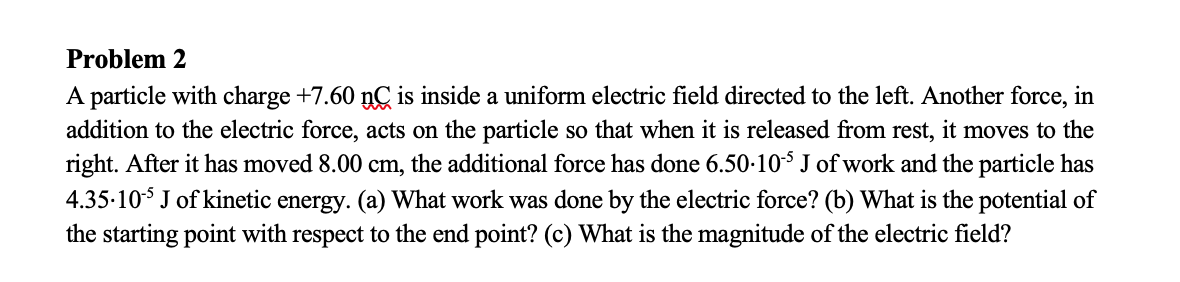Problem 2 A particle with charge +7.60 nC is inside a uniform electric field directed to the left. Another force, in addition to the electric force, acts on the particle so that when it is released from rest, it moves to the right. After it has moved 8.00 cm, the additional force has done 6.50-10-4 J of work and the particle has 4.35-10-5 J of kinetic energy. (a) What work was done by the electric force? (b) What is the...

• ### Please complete both The electric field strength between two parallel conducting plates separated by 4.20 cm...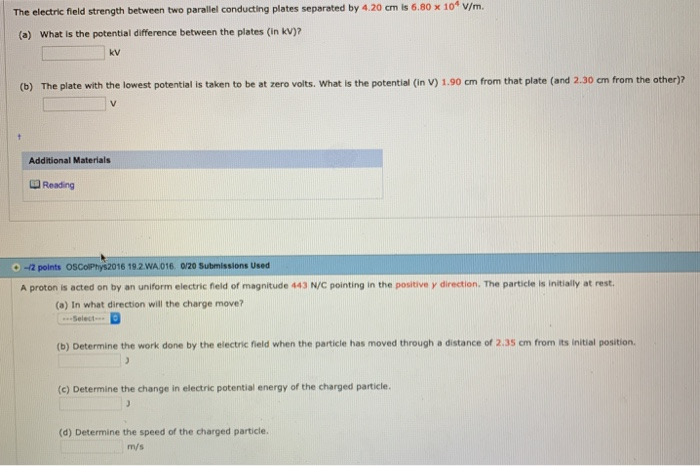Please complete both The electric field strength between two parallel conducting plates separated by 4.20 cm is 6.80 x 104 v/m. (a) What is the potential difference between the plates (in kV)2 kv (b) The plate with the lowest potential is taken to be at zero volts. What is the potential (in V) 1.90 cm from that plate (and 2.30 cm from the other)? Additional Materials Reading .-2 points oscoPhys2016 19.2.WA016 0/20 Submissions Used A proton is acted on by...

• ### A uniform electric field of magnitude 280 V/m is directed in the positive x direction. A...

A uniform electric field of magnitude 280 V/m is directed in the positive x direction. A +15.0 µC charge moves from the origin to the point (x, y) = (20.0 cm, 50.0 cm). (a) What is the change in the potential energy of the charge field system? J (b) Through what potential difference does the charge move? V

• ### A uniform electric field of magnitude 250 V/m is directed in the positive x direction. A...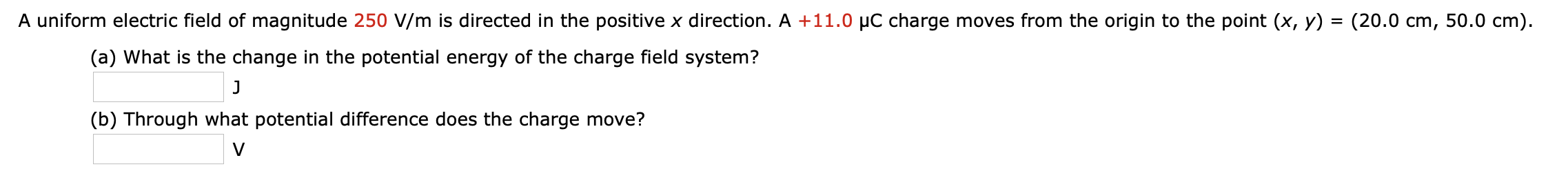A uniform electric field of magnitude 250 V/m is directed in the positive x direction. A +11.0 PC charge moves from the origin to the point (x, y) = (20.0 cm, 50.0 cm). (a) What is the change in the potential energy of the charge field system? (b) Through what potential difference does the charge move?

• ### A uniform electric field of magnitude 211 V/m is directed in the positive x direction. A...

A uniform electric field of magnitude 211 V/m is directed in the positive x direction. A -13.5 μC charge moves from the origin to the point (x, y) = (21.7 cm, 54.1 cm). What was the change in the potential energy of this charge? Through what potential difference did the charge move?

• ### astatements about electric potential and electric potential energy is/are correct? he electric potential energy ap...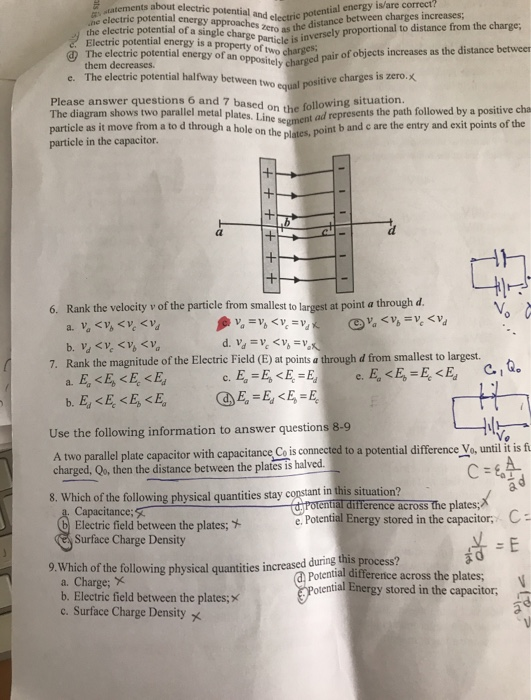astatements about electric potential and electric potential energy is/are correct? he electric potential energy approaches zero as the distance between charges increases; the electric potential of a single charge particle is inversely proportional to distance from the charge, Electric potential energy is a property of two charges The electric potential energy of an oppositely charged pair of objects increases as the distance betweem e. The electric potential halfway between two equal positive charges is zero.x them decreases. Please answer questions...